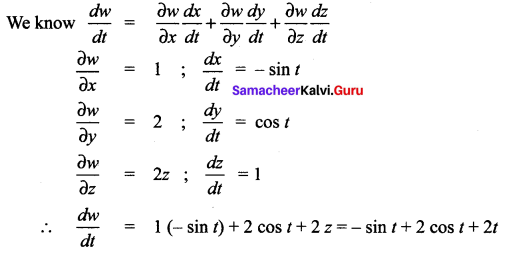## Tamilnadu Samacheer Kalvi 12th Maths Solutions Chapter 8 Differentials and Partial Derivatives Ex 8.6

Question 1.
If u(x, y) = x2y + 3xy4, x = et and y = sin t, find $$\frac{d u}{d t}$$ and evaluate it at t = 0.
Solution: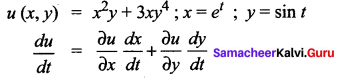= (2xy + 3y4) (et) + (x2 + 12xy3) (cos t)
= (2et sin t + 3 sin4 t) et + [e2t + 12et sin3 t] cos t
= et [2et sin t + 3 sin4 t + et (cos t) + 12 sin3t cos t]
at t = 0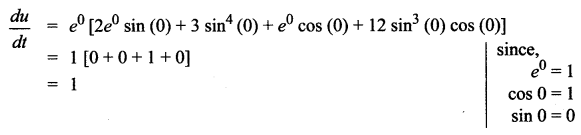Question 2.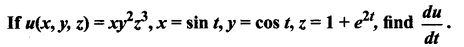Solution: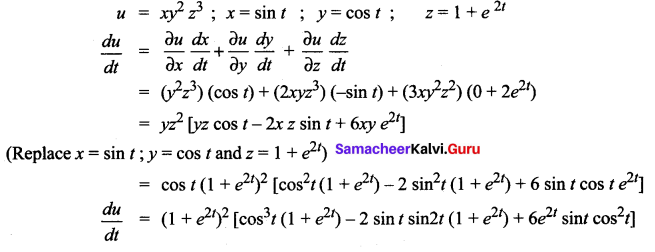Question 3.
If w(x, y, z) = x2 + y2 + z2, x = et, y = et sin t, and z = etcos t, find $$\frac{d w}{d t}$$
Solution:
w(x, y, z) = x2 + y2 + z2 ; x = et ; y = et sin t, z = et cos t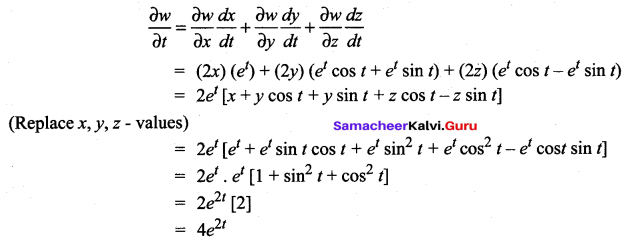Question 4.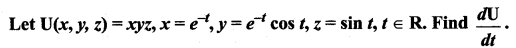Solution: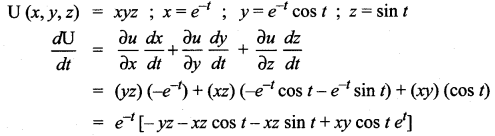(Replace x, y, z value)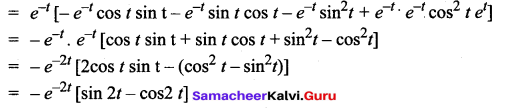Question 5.
If w(x, y) = 6x3 – 3xy + 2y2, x = es, y = cos s, s ∈ R, find $$\frac{d w}{d s}$$, and evaluate at s = 0.
Solution: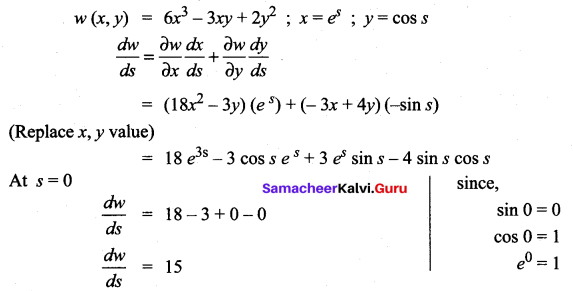Question 6.
If z(x, y) = x tan-1 (x y), x = t2, y = s et, s, t ∈ R, Find $$\frac{\partial z}{\partial \mathbf{t}}$$ and $$\frac{\partial z}{\partial \mathbf{t}}$$ at s = t = 1
Solution:
z (x, y) = x tan-1 (xy) ; x = t2 ; y = set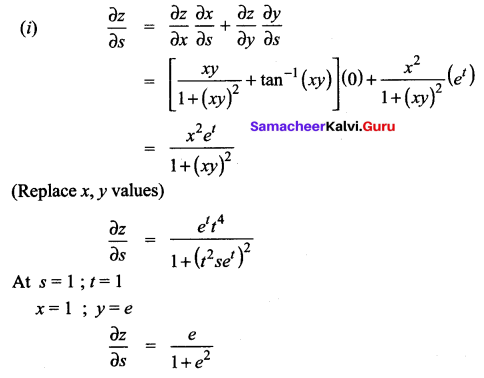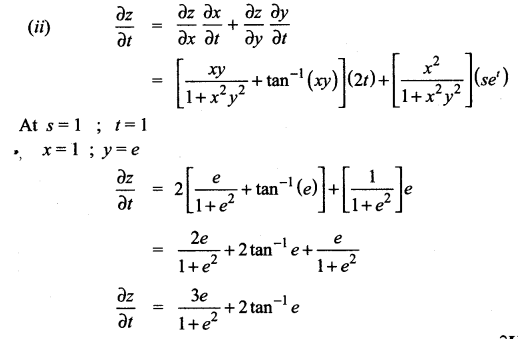Question 7.
Let U (x, y) = ex sin y, where x = st2, y = s2t, s, t ∈ R. Find them at s = t = 1.
Solution: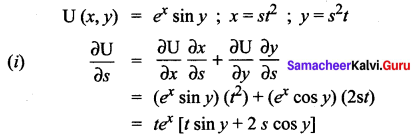(Replace x, y values)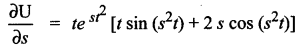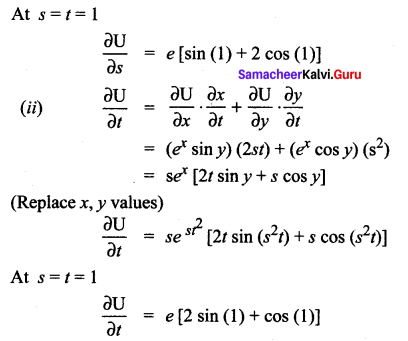Question 8.
Let z(x, y) = x3 – 3x2y3, where x = set, y = se-t, s, t ∈ R. Find $$\frac{\partial z}{\partial s}$$ and $$\frac{\partial z}{\partial t}$$
Solution: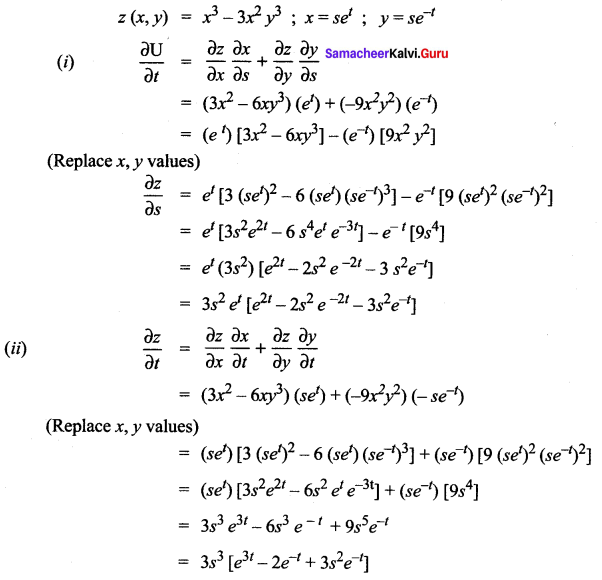Question 9.
W(x,y, z) = xy + yz + zx, x = u -v, y = uv, z = u + v, u, v e R. Find $$\frac{\partial \boldsymbol{w}}{\partial \boldsymbol{u}}$$, $$\frac{\partial \boldsymbol{w}}{\partial \boldsymbol{v}}$$ them at $$\left(\frac{1}{2}, 1\right)$$
Solution: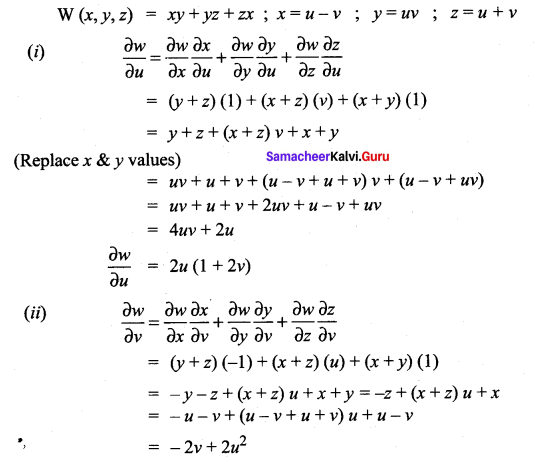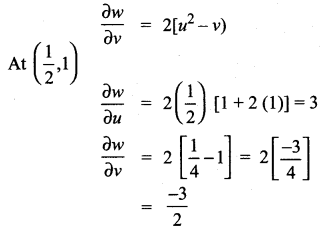### Samacheer Kalvi 12th Maths Solutions Chapter 8 Differentials and Partial Derivatives Ex 8.6 Additional Problems

Question 1.
Suppose that z = $$y e^{x^{2}}$$ where x = 2t and y = 1 – t then find $$\frac{d z}{d t}$$.
Solution: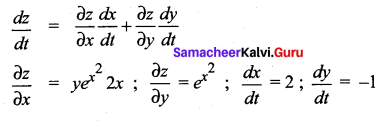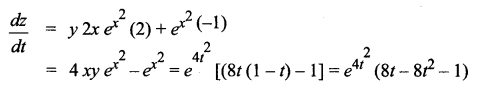(Since x = 2t and y = 1 – t)Question 2.
If w = x + 2y + z2 and x = cos t ; y = sin t ; z = t. Find $$\frac{d w}{d t}$$.
Solution: